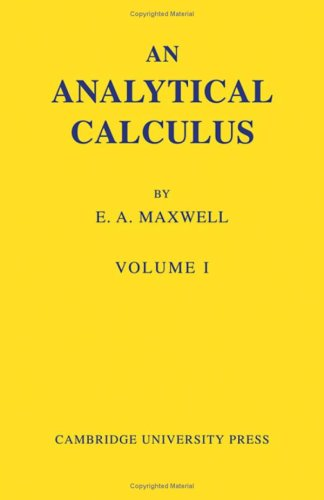Free Shipping in Australia# An Analytical Calculus by E. A. Maxwell

In Stock
\$22.49
inc. GST
This is the first volume of a series covering all stages of development of the Calculus, from the last year at school to degree standard. This volume is concerned with the basic ideas and applications of differentiation and integration in relation to algebraic and trigonometric functions, but excluding logarithmic and exponential functions.
Only 1 left

## An Analytical Calculus Summary

### An Analytical Calculus: Volume 1 by E. A. Maxwell

This is the first volume of a series of four volumes covering all stages of development of the Calculus, from the last year at school to degree standard. The books are written for students of science and engineering as well as for specialist mathematicians, and are designed to bridge the gap between the works used in schools and more advanced studies, with their emphasis on rigour. This volume is concerned with the basic ideas and applications of differentiation and integration in relation to algebraic and trigonometric functions, but excluding logarithmic and exponential functions. Integration begins on the 'Riemann integral' basis, and the treatment of curves combines accuracy with simplicity, without shirking the awkward problems of sign. Each section has examples; at the end of each chapter there are problems from school-leaving and open scholarship examinations.

### Why buy from World of BooksOur excellent value books literally don't cost the earthFree delivery in AustraliaEvery used book bought is one saved from landfill

Preface; Introduction; 1. The idea of differentiation; 2. The evaluation of differential coefficients; 3. Applications of differentiation; 4. The idea of integration; 5. Devices in integration; 6. Applications of integration; Answers to examples; Index.

GOR010733411
An Analytical Calculus: Volume 1 by E. A. Maxwell
E. A. Maxwell